# Chapter 3 practice question answers

3.1. Introduction to Functions

1. Yes

2. No

3. Yes

4. Q = g(4) = 6

5. x = 0 or x = 2

6. a. g(5) = 1

b. m = 8

3.2. Domain and Range

1. Domain; y = years [1960,2010] ; Range, p = population, [100,1400]

2. a. Values that are less than or equal to -2, or values that are greater than or equal to -1 and less than 3

b. {x | x ≤ -2 or -1 ≤ x < 3}

c. (-∞, -2] ∪ [-1, 3)

3. Domain; y = years, [1952,2002] ; Range, p = population in millions, [40,88]

4.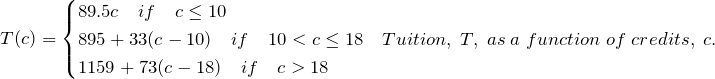A reasonable domain should be whole numbers 0 to (answers may vary), e.g. [0,23]. A reasonable range should be $0 – (answers may vary), e.g. [0,1524]. 5. a. {x | 2008 ≤ x ≤ 2018}, [2008, 2018] b. {x | 16.1 ≤ x ≤ 88.4}, [16.1, 88.4] 3.3. Rates of Change and Behaviour of Graphs 1.$0.264 dollars per year.

2. Average rate of change =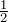.

3.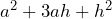4. Based on the graph, the local maximum appears to occur at (-1, 28), and the local minimum occurs at (5,-80). The function is increasing on (-∞, -1)∪(5, ∞) and decreasing on (-1, 5).

5.  a. -0.59 per 100,000

b. 6

c. 2010, 2014, and 2017

1.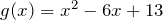in standard form;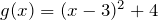in vertex form.

2. a. Vertex is a minimum value (opens upwards).

b. Vertex is a maximum value (opens downwards).

3.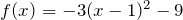4. Two sides with lengths of 8 m and the longer side with a length of 16 m will give a maximum dimension of 128 m2.

5. \$5387.50

6. a.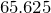m

b.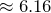sec